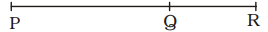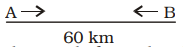## Speed, Time and Distance

#### Speed, Time and Distance

1. A man travelled a distance of 61 km in 9 hours, partly on foot at the rate of 4 km/hr and partly on bicycle at the rate of 9 km/hr. The distance travelled on foot was
1. 12 km
2. 16 km
3. 20 km
4. 24 km

1. Let man walked for t hours.
then, t × 4 + (9 – t) × 9 = 61
⇒  4t + 81 – 9t = 61
⇒  81 – 5t = 61
⇒  5t = 20
⇒  t = 4
∴  Distance travelled on foot
= 4 × 4 = 16 km.

##### Correct Option: B

Let man walked for t hours.
then, t × 4 + (9 – t) × 9 = 61
⇒  4t + 81 – 9t = 61
⇒  81 – 5t = 61
⇒  5t = 20
⇒  t = 4
∴  Distance travelled on foot
= 4 × 4 = 16 km.

1. Ravi and Ajay start simultaneously from a place A towards B, 60 km apart. Ravi’s speed is 4 km/hr less than that of Ajay. Ajay, after reaching B, turns back and meets Ravi at a place 12 km away from B. Ravi’s speed is
1. 12 km/hr
2. 10 km/hr
3. 8 km/hr
4. 6 km/hr

1. Let the speed of Ravi be x kmph
then, Ajay’s speed = (x + 4) kmph
Distance covered by Ajay
= 60 + 12 = 72 km
Distance covered by Ravi
= 60 – 12 = 48 km.
According to the question,

 72 = 48 x + 4 x

 ⇒ 3 = 2 x + 4 x

⇒  3x = 2x + 8
⇒  x = 8 kmph

##### Correct Option: C

Let the speed of Ravi be x kmph
then, Ajay’s speed = (x + 4) kmph
Distance covered by Ajay
= 60 + 12 = 72 km
Distance covered by Ravi
= 60 – 12 = 48 km.
According to the question,

 72 = 48 x + 4 x

 ⇒ 3 = 2 x + 4 x

⇒  3x = 2x + 8
⇒  x = 8 kmph

1. P and Q are 27 km away. Two trains with speed of 24 km/hr and 18 km/hr respectively start simultaneously from P and Q and travel in the same direction. They meet at a point R beyond Q. Distance QR is
1. 126 km
2. 81 km
3. 48 km
4. 36 km

1.Let the trains meet after t hours, then
24t – 18t = 27
⇒  6t = 27

 ⇒  t = 27 6

 = 9 hours 2

 ∴ QR = 18t = 18 × 9 = 81 km 2

##### Correct Option: BLet the trains meet after t hours, then
24t – 18t = 27
⇒  6t = 27

 ⇒  t = 27 6

 = 9 hours 2

 ∴ QR = 18t = 18 × 9 = 81 km 2

1. From two places, 60 km apart, A and B start towards each other at the same time and meet each other after 6 hours.
 Had A travelled with 2 of his speed 3
and B travelled with double of his speed, they would have met after 5 hours. The speed of A is
1. 4 km/hr.
2. 6 km/hr.
3. 10 km/hr.
4. 12 km/hr.

1.Let the speed of A = x kmph and that of B = y kmph
According to the question,
x × 6 + y × 6 = 60
⇒  x + y = 10    ....(i)

 and, 2 x × 5 + 2y × 5 = 60 3

⇒  10x + 30y = 180
⇒  x + 3y = 18    ...(ii)
From equations (i) × (3) – (ii)
3x + 3y – x – 3y = 30 – 18
⇒  12x = 12
⇒  x = 6 kmph.

##### Correct Option: BLet the speed of A = x kmph and that of B = y kmph
According to the question,
x × 6 + y × 6 = 60
⇒  x + y = 10    ....(i)

 and, 2 x × 5 + 2y × 5 = 60 3

⇒  10x + 30y = 180
⇒  x + 3y = 18    ...(ii)
From equations (i) × (3) – (ii)
3x + 3y – x – 3y = 30 – 18
⇒  12x = 12
⇒  x = 6 kmph.

1. A man completed a certain journey by a car. If he covered 30% of the distance at the speed of 20km/hr, 60% of the distance at 40km/hr and the remaining distance at 10km/hr; his average speed for the whole journey was
1. 25 km/hr
2. 28 km/hr
3. 30 km/hr
4. 33 km/hr

1. Let the total distance be 100 km.

 Average speed = Total distance covered Time taken

 = 100 3 + 3 + 1 2 2

 = 100 3 + 3 + 2 2

 = 100 × 2 = 25 kmph 8

##### Correct Option: A

Let the total distance be 100 km.

 Average speed = Total distance covered Time taken

 = 100 3 + 3 + 1 2 2

 = 100 3 + 3 + 2 2

 = 100 × 2 = 25 kmph 8